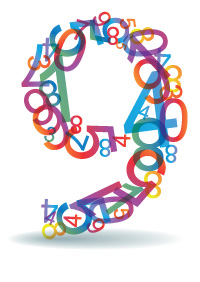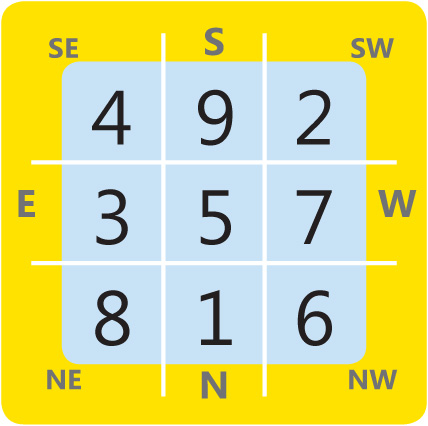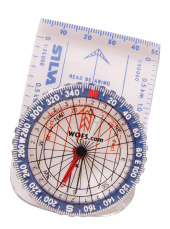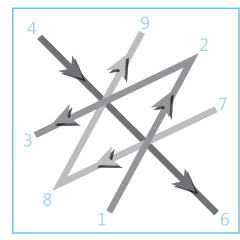Why the Number 9 is the Most Supreme of NumbersWOFS is delighted to introduce David Burton, mathematical genius and very successful Astrologer from Australia. David and his Russian wife the lovely Elena, who is herself an expert in medieval astrology were in Kuala Lumpur recently to learn feng shui, and in the course of the week long Master Practitioners Program, impressed us all with the mathematical co-relations he picked up instantly from all the formulas used in feng shui. In this feature on the magic of numbers, David walks us through the vital significance of the auspicious numbers and how they are “hidden” beneath the numbers of the Lo Shu square and in the compass of directions – two vital tools of feng shui, in particular the supreme whole number – 9!

The Master Number 9 is an important number signifying wholeness and completion exactly as Lillian has taught, and while attending the recent MPC in feng shui in Malaysia, I also discovered that all the angles and “degrees” of the compass, the flight of the “stars” in the Lo Shu chart with 5 in the centre that is the basis of all flying star charts expressed as numbers in  the three by three grid… all added up to 9!

Not surprising as 9 is the highest digit before we go back to 1.The Lo Shu square in Feng Shui has nine numbers placed in an arrangement with each number in each grid so that any three numbers in a straight line – whether horizontally, vertically or diagonally add up to 15, which as Lillian explained is the number of days it takes the moon to reach fullness. It doesn’t matter which way you add the numbers they add to 15. The Lo Shu square is the magic square of three. It is also the square of the planet Saturn, so 3X3 =9! Feng Shui definitely has a correlation to astrology, which I see as the basis of all systems, and this can be proven mathematically.

Note that when you add up all the number  1 to 9 i.e. 1+2+3+4+5+6+7+8+9  they add up to 45 degrees, representing each major direction on the compass, and here we see that once again  4+5 also adds up to 9.

The 9 hidden in the COMPASS and the Feng Shui formulasThe number 9 transcends the feng shui compass, and all the formulas of feng shui thus have the 9 hidden in each of the direction houses. Take the Eight Mansions formula for instance. According to this formula, there are eight directions major direction categories and these are 45 degrees each. Eight times forty-five equals three hundred and sixty degrees which is one circle or a complete cycle. Note that all the important 45 degree angles add up to nine. One eighth = 45degrees, (4+5=9), one quarter = 90 degrees (9+0=9), three eights = 135 degrees (1+3+5=9), one half = 180 degrees (1+8+0=9), five eights = 225 degrees (2+2+5=9), three quarters = 270 degrees (2+7+0=9), seven eights = 315 degrees (3+1+5=9) and the last comes back to 360 degrees (3+6+0 =9). So all the important angles add up to 9! This suggests that 9 is the most important number in feng shui formulas that use the compass.

Connection to STOCK MARKET

The Chinese agricultural (HSIA or solar) calendar starts on the 4th February each year; the 4th February on the astrological calendar is 315 degrees and this too adds up to the number nine. The astrological year starts on the 21st March each year and if you add 45 degrees to the starting point of zero degrees Aries you end up with the following eight dates which are important turning points in the stock markets, commodities and currencies.

NOTE THESE DATES – they are turning points for the financial markets. We have underlined the dates that are coming soon.

The 6th May (45 degrees),22nd June (90 degrees), 8th August (135 degrees),22nd September (180 degrees), 7th November (225 degrees), 22nd December (270 degrees), 4th February (315 degrees) and back to the beginning again completing the circle or cycle.

FLIGHT OF THE STAR NUMBERS IN THE LO SHU SQUARE…i.e. moving alternately down and up

The way the numbers fly in the Lo Shu square (i.e. the flight pattern) also transcends the number 9. Thus note that the number 5 moving down 135 degrees to 6 in the NW, then up 180 degrees to 7 in the West pattern, then down 45 degrees across to 8 the Northeast and so forth – the angle of flight of the numbers going from one to nine move in angles of  135, 135, 45,180,45,135 and 135, and every angle of degrees adds up to 9. Even when these total angles in degrees are added together, they make a sum of 810, which again add up to 9. Not just that, but drop the zero from 810 and you have 81, which is the square of nine. This stands for the moon, and the moon of course is always related to the passage of time i.e. to the calendar! I am thus not surprised when Lillian tells us that the Lo Shu square holds many secrets, and that the square unlocks the change of energy over time.There are other magic squares with one up to nine in the centre that relate to the other planets and numbers. This is also reflected in the number of squares per side; so the “square” is sequentially enlarged. The reason Feng Shui uses the magic square of three or which belongs to the planet Saturn, is that Saturn stands for real-estate or home and feng shui is about improving the home, so that it is in harmony with the cycles of a home’s life.

The square of the numbers 1 and 6 is for the Sun, while the magic square of  6, 2 and 7 are for the moon; these two numbers are added together to make the magic square of 9 for the moon. 3 is for Saturn which is also the Lo Shu square, 4 is the magic square of Jupiter,5 is the magic square of Mars ,7 is the magic square of Venus and 8 is the magic square of Mercury.  Lillian explained that these “magic squares” are also used in advanced feng shui.

The 12 animals and the Five Elements in Feng Shui

The earth rotates 15 degrees every hour and 24 hours x 15 = 360 or 1 day. The 24 Mountains relate to the 15 degree movement of the earth. Every year Jupiter moves 15 degrees and the is where the twelve animals comes from in Chinese astrology and Feng Shui. The elements run Earth, Mental, Water, Wood and Fire. These elements are 72 degrees apart. The 5 pointed star that is the symbol of the 5 elements is also the symbol of the Vitruvian Man. The pattern of the five pointed star starts at zero which has to start in the earth sign at 4th February and moves 144 degrees times five which equals a movement of 720 degrees to get back to zero.